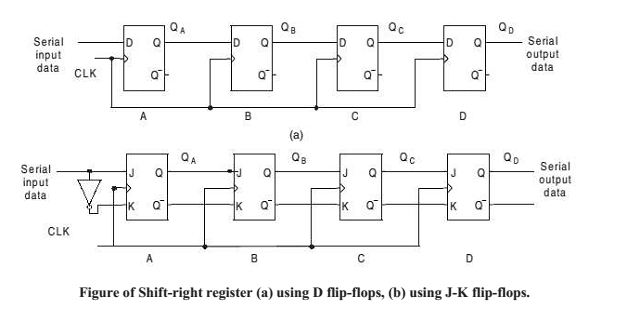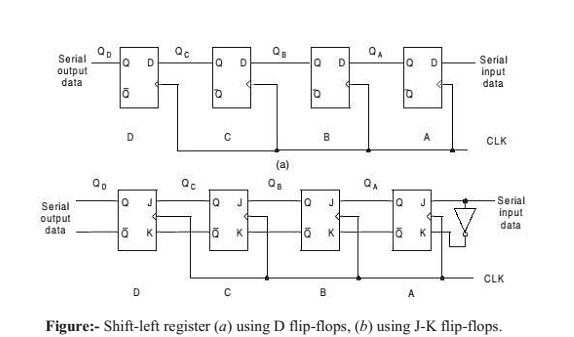REGISTERS

A register is a group of binary storage cells capable of holding binary information. A group of flip-flops constitutes a register, since each flip-flop can work as a binary cell. An n-bit register, has n flip-flops and is capable of holding n-bits information. In addition to flip-flops a register can have a combinational part that performs data-processing tasks.

Register:

•  A set of n flip-flops
•  Each flip-flop stores one bit
•  Two basic functions: data storage and data movement.

Shift Register: A register that allows each of the flip-flops to pass the stored information to its adjacent neighbor.

Counter: A register that goes through a predetermined sequence of states.

Storage Capacity of a register

The storage capacity of a register is the total number of bits (1 or 0) of digital data it can retain. Each stage (flip flop) in a shift register represents one bit of storage capacity. Therefore the number of stages in a register determines its storage capacity.

Shift Register

A shift register is a storage device that used to store binary data. When a number of flip flop are connected in series it is called a register. A single flip flop is supposed to stay in one of the two stable states 1 or 0 or in other words the flip flop contains a number 1 or 0 depending upon the state in which it is. A register will thus contain a series of bits which can be termed as a word or a byte.

If in these registers the connection is done in such a way that the output of one of the flip flop forms in input to other, it is known as a shift register. The data in a shift register is moved serially (one bit at a time).

The shift register can be built using RS, JK or D flip-flops various types of shift registers are available some of them are given as under.

• 1. Shift Left Register
• 2. Shift Right Register
• 3. Shift Around Register
• 4. Bi-directional Shift Register

There are two ways to shift data into a register (serial or parallel) and similarly two ways to shift the data out of the register. This leads to the construction of four basic types of registers:-

• 1. Serial in/Serial out (SISO)
• 2. Serial in/Parallel out (SIPO)
• 3. Parallel in/Serial out (PISO)
• 4. Parallel in/Parallel out (PIPO

SERIAL-IN–-SERIAL-OUT SHIFT REGISTER

From the name itself it is obvious that this type of register accepts data serially, i.e., one bit at a time at the single input line. The output is also obtained on a single output line in a serial fashion.

The data within the register may be shifted from left to right using shift-left register, or may be shifted from right to left using shiftright register.

Shift-right Register

A shift-right register can be constructed with either J-K or D flip-flops as shown in Figure 8.3. A J-K flip-flop based shift register requires connection of both J and K inputs. Input data are connected to the J and K inputs of the left  most (lowest order) flip-fl op. To input a 0, one should apply a 0 at the J input, i.e., J = 0 and K = 1 and vice versa.

With the application of a clock pulse the data will be shifted by one bit to the right. In the shift register using D flip-flop, D input of the left most flip-flop is used as a serial input line. To input 0, one should apply 0 at the D input and vice versa.The clock pulse is applied to all the flip-flops simultaneously. When the clock pulse is applied, each flip-flop is either set or reset according to the data available at that point of time at the respective inputs of the individual flip-flops. Hence the input data bit at the serial input line is entered into flip-flop A by the first clock pulse.

At the same time, the data of stage A is shifted into stage B and so on to the following stages. For each clock pulse, data stored in the register is shifted to the right by one stage. New data is entered into stage A, whereas the data present in stage D are shifted out (to the right).

Shift-left Register
A shift-left register can also be constructed with either J-K or D flip-flops as shown in Figure below. Let us now illustrate the entry of the 4-bit number 1110 into the register, beginning with the right-most bit. A 0 is applied at
the serial input line, making D = 0. As the first clock pulse is applied, flip-fl op A is RESET, thus storing the 0.Next a 1 is applied to the serial input, making D = 1 for flip-flop A and D = 0 for flip-flop B, because the input of flip-flop B is connected to the QA output.

When the second clock pulse occurs, the 1 on the data input is “shifted” to the flip-flop A and the 0 in the flipflop A is “shifted” to flip-flop B. The 1 in the binary number is now applied at the serial input line, and the third clock pulse is now applied. This 1 is entered in flip-flop A and the 1 stored in flip-flop A is now “shifted” to flip-flop B and the 0 stored in flip-flop B is now “shifted” to flip-flop C.

The last bit in the binary number that is the 1 is now applied at the serial input line and the fourth clock pulse is now applied. This 1 now enters the flipflop A  and the 1 stored in flip-flop A is now “shifted” to flip-flop B and the 1 stored in flip-flop B is now “shifted” to flip-flop C and the 0 stored in flip-flop C is now “shifted” to flip-flop D. Thus the entry of the 4-bit binary number in the shift-right register is now completed.8-bit Serial-in–Serial-out Shift Register

The pinout and logic diagram of IC 74L91 is shown in Figure below. This IC is actually an example of an 8-bit serial-in–serial-out shift register. There are eight S-R flip-flops connected to provide a serial input as well as a serial output. The clock input at each flip-flop is negative edge-triggered.

However, the applied clock signal is passed through an inverter. Hence the data will be shifted on the positive edges of the input clock pulses. An inverter is connected in between R and S on the first flip-flop. This means that this circuit functions as a Dtype flip-flop. So the input to the register is a single liner on which the data can be shifted into the register appears serially. The data input is applied at either A (pin 12) or B (pin 11).

The data level at A (or B) is complemented by the NAND gate and then applied to the R input of the first flip-flop. The same data level is complemented by the NAND gate and then again complemented by the inverter before it appears at the S input. So, a 0 at input A will reset the first flip-flop (in other words this 0 is shifted into the first flip-flop) on a positive clock transition.
The NAND gate with A and B inputs provide a gating function for the input data stream if required, if gating is
not required, simply connect pins 11 and 12 together and apply the input data stream to this connection.A is held high: The NAND gate is enabled and the serial input data passes through the NAND gate inverted.
The input data is shifted serially into the register.

A is held low: The NAND gate output is forced high, the input data steam is inhibited, and the next clock pulse
will shift a 0 into the first flip-flop. Each succeeding positive clock pulse will shift another 0 into the register.
After eight clock pulses, the register will be full of zeros.

PARALLEL-IN–SERIAL-OUT REGISTER
In the preceding two cases the data was shifted into the registers in a serial manner. Here we develop an idea for
the parallel entry of data into the register. Here the data bits are entered into the flip-flops simultaneously, rather
than a bit-by-bit basis

8-bit Parallel-in–Serial-out Shift Register
The data can be loaded into the register in parallel and shifted out serially at QH using either of two clocks (CLK or CLK inhibit). It also contains a serial input, DS through which the data can be serially shifted in.

PARALLEL-IN–PARALLEL-OUT REGISTER

The parallel input of data has already been discussed in the preceding section of parallel-in–serial-out shift register. Also, in this type of register there is no interconnection between the flip-flops since no serial shifting is required. Hence, the moment the parallel entry of the data is accomplished the data will be available at the parallel outputs of the register.

UNIVERSAL REGISTER

A register that is capable of transferring data in only one direction is called a ‘unidirectional shift register’ whereas the register that is capable of transferring data in both left and right direction is called a ‘bidirectional shift register’. Now if the register has both the shift-right and shift-left capabilities, along with the necessary input and output terminals for parallel transfer, then it is called a shift register with parallel load or ‘universal shift register’.

The most general shift register has all the capabilities listed below. Others may have only some of these functions, with at least one shift operation.

• 1) A shift-right control to enable the shift-right operation and the serial input and output lines associated with
the shift-right.
• 2) A shift-left control to enable the shift-left operation and the serial input and output lines associated with the
shift-left.
• 3) A parallel-load control to enable a parallel transfer and the n input lines associated with the parallel transfer.
• 4) n parallel output lines.
• 5) A clear control to clear the register to 0.
• 6) A CLK input for clock pulses to synchronize all operations.
• 7) A control state that leaves the information in the register unchanged even though clock pulses are continuously applied.

SHIFT REGISTER COUNTERS

Shift registers may be arranged to form different types of counters. These shift registers use feedback, where the output of the last flip-flop in the shift register is fed back to the first flip-flop.

Based on the type of this feedback connection, the shift register counters are classified as (i) ring counter and (ii) twisted ring or Johnson or Shift counter.

Asynchronous and Synchronous Shift Registers

Asynchronous circuits changes state each time the input changes the state, while synchronous circuit changes state only when triggered by a momentary change in the input signal. This momentary change is called triggering. Shift registers are made of flip flops and their operation depends upon the state at the flip flops.

Flip flops changes their states due to triggering when flip flop change their state on the base of input pulse then it is called Edge triggering.

In edge triggering flip flop change its state on the basses of Leading edge or trailing edge. When flip flop works on the bases of change in DC level, that is called Asynchronous Triggering. And the shift registers work on this principle is called Asynchronous shift registers. On the other hand, shift registers changes their state only when triggered by clock pulse are called Synchronous shift registers these type of shift registers usually used in counters.

You may be interested in:
Digital Electronics MCQs